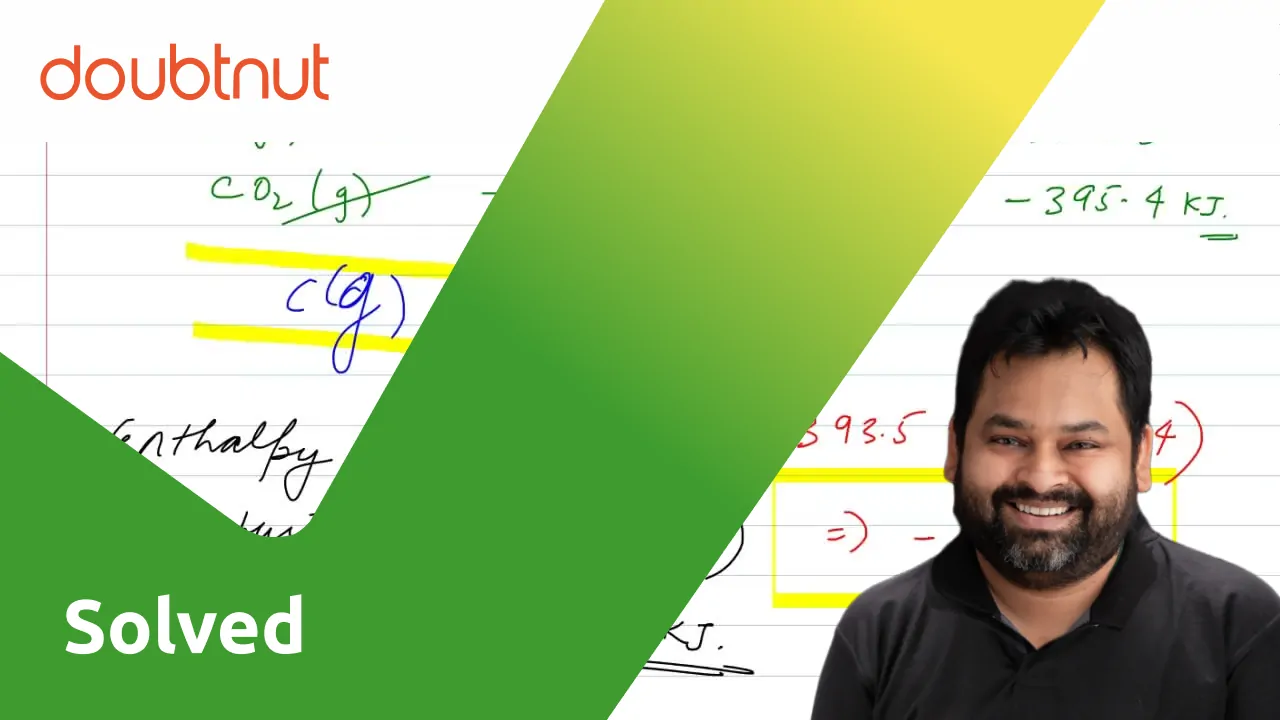Doubtnut App - Get 50 Lakh+ video solutions better than GoogleInstall Now

# Calculate the enthalpy change accompanying the transformation of C(graphite) to C(diamond). Given that the enthalpies of combustion of graphite and diamond are 393.5 and 395.4 KJ respectively

Text Solution
Verified by Experts

## We are given (i) C (graphite) +O2(g)→CO2(g),ΔcH∘=−393.5kJmol−1 (ii) C (diamond) +O2(g)→CO2(g),ΔcH∘=−395.4kJmol−1 We aim at C( graphite ) → C ( diamond) ,ΔtransH∘=? Subtracting eqn. (ii) from eqn. (i), we get or C(graphite)- C (diamond) →0,ΔrH∘=−393.5−(−395.4)=+1.9kJ